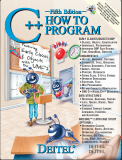Back to www.deitel.comISBN: 0-13-185757-6 © 2005 pages: 1500 Buy the Book!This tutorial introduces C++ exception handling. An exception is an indication of a problem that occurs during a program's execution. The name "exception" implies that the problem occurs infrequently—if the "rule" is that a statement normally executes correctly, then the "exception to the rule" is that a problem occurs. The techniques presented in this tutorial enable programmers to write robust, fault-tolerant programs that are able to deal with problems that may arise, and continue executing or terminate gracefully. We demonstrate exception-handling techniques with an example in which a function generates an exception when an attempt is made to divide by zero. The program catches this exception, issues an error message, then continues normal execution. This tutorial is intended for students and professionals who are already familiar with building C++ classes.

Download the code examples for this tutorial.

[Note: This tutorial is an excerpt (Section 16.3) of Chapter 16, Exception Handling, from our textbook C++ How to Program, 5/e. These tutorials may refer to other chapters or sections of the book that are not included here. Permission Information: Deitel, Harvey M. and Paul J., C++ HOW TO PROGRAM, ©2005, pp.812-818. Electronically reproduced by permission of Pearson Education, Inc., Upper Saddle River, New Jersey.]

### 16.3 Example: Handling an Attempt to Divide by Zero

Let us consider a simple example of exception handling (Fig. 16.1–Fig. 16.2). The purpose of this example is to prevent a common arithmetic problem—division by zero. In C++, division by zero using integer arithmetic typically causes a program to terminate prematurely. In floating-point arithmetic, division by zero is allowed—it results in positive or negative infinity, which is displayed as `INF` or `-INF`.

In this example, we define a function named `quotient` that receives two integers input by the user and divides its first `int` parameter by its second `int` parameter. Before performing the division, the function casts the first `int` parameter’s value to type `double`. Then, the second `int` parameter’s value is promoted to type `double` for the calculation. So function `quotient` actually performs the division using two `double` values and returns a `double` result.

Although division by zero is allowed in floating-point arithmetic, for the purpose of this example, we treat any attempt to divide by zero as an error. Thus, function `quotient` tests its second parameter to ensure that it is not zero before allowing the division to proceed. If the second parameter is zero, the function uses an exception to indicate to the caller that a problem occurred. The caller (`main` in this example) can then process this exception and allow the user to type two new values before calling function `quotient` again. In this way, the program can continue to execute even after an improper value is entered, thus making the program more robust.

The example consists of two files—`DivideByZeroException.h` (Fig. 16.1) defines an exception class that represents the type of the problem that might occur in the example, and `fig16_02.cpp` (Fig. 16.2) defines the `quotient` function and the `main` function that calls it. Function `main` contains the code that demonstrates exception handling.

Additional Pages in this Tutorial: Page 1 | 2 | 3 | 4
Tutorial Index